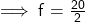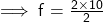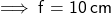## The radius of curvature of a spherical mirror is 20cm.What is its focal length?

Question

The radius of curvature of a spherical mirror is 20cm.What is its focal length?

in progress 0
2 months 2021-08-03T16:43:15+00:00 2 Answers 2 views 0

1. Focal length = 10 cm.

Solution :

Given radius of curvature = 20 cm.

Formula :

Focal length = Radius of curvature/2

→ Focal length = 20/2

→ Focal length = 10 cm.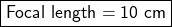Given:

Radius of curvature (R) of a spherical mirror = 20

To Find:

Focal length (f)

Explanation:

Formula: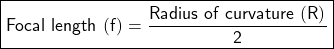Substituting value of R in the equation: